# Class 6 Maths NCERT Solutions for Chapter 7 Fractions Ex – 7.4

## Fractions

Question 1.
Write shaded portion as fraction. Arrange them in ascending and descending order using correct sign ‘>’, ‘=’, ‘>’ between the fractions: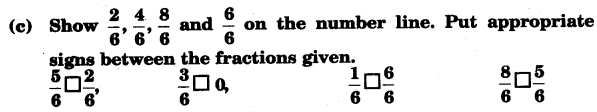Solution: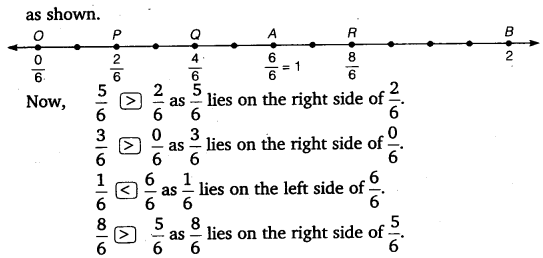Question 2.
Compare the fractions and put an appropriate sign.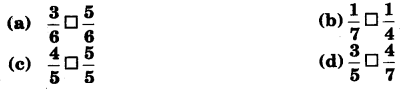Solution: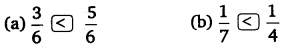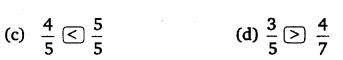Question 3.
Make five more such pairs and put appropriate signs.

Solution:
Five more such pairs may be taken as under:
Compare the fractions and put an appropriate sign.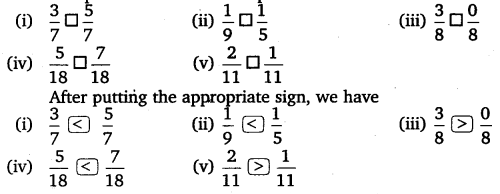Question 4.
Look at the figures and write ‘<’ or ‘>’, ‘=’ between the given pairs of fractions.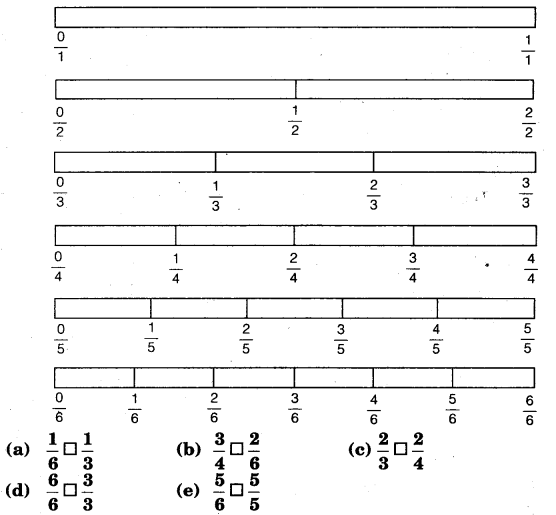Make five more such problems and solve them with your friends.

Solution: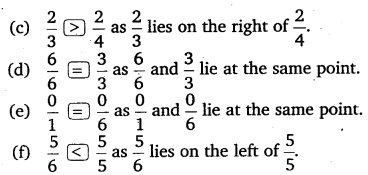Question 5.
Five more such problems are as under:

Look at the figures given in question 5 and write ‘<’ or ‘>’ or ‘=’ between the pairs of fractions.Solution:Question 6.
How quickly can you do this? Fill appropriate sign. (<, =, >)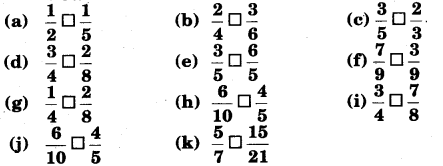Solution: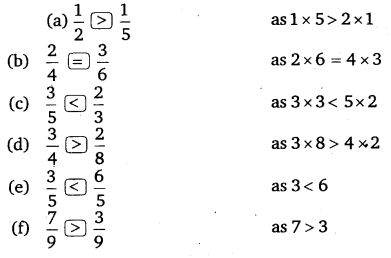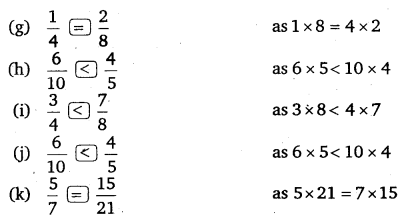Question 7.
The following fractions represent just three different numbers. Separate them into three groups of equivalent fractions, by changing each one to its simplest form.Solution: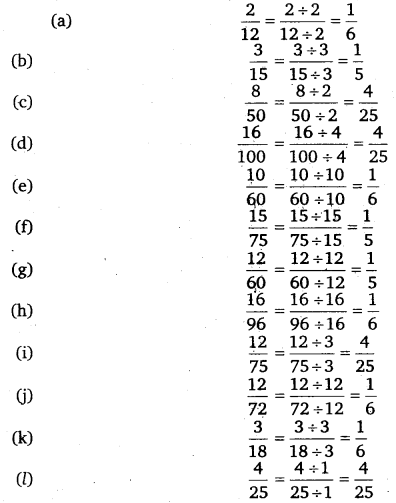On separating them into three groups of equivalent fractions, we haveQuestion 8.
Find answers to the following. Write and indicate how you solved them.Solution:Question 9.
Ila read 25 pages of a book containing 100 pages. Lalita read$\frac { 2 }{ 5 }$ of the same book. Who read less?

Solution:Question 10.
Rafiq exercised for$\frac { 3 }{ 6 }$ of an hour, while Rohit exercised for$\frac { 3 }{ 4 }$ of
an hour. Who exercised for a longer time?

Solution: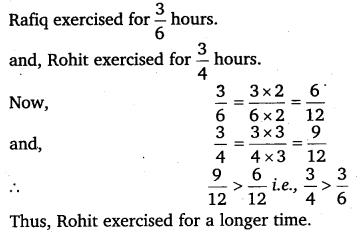Question 11.
In a class A of 25 students, 20 passed in first class; in another class B of 30 students, 24 passed in first class. In which class was a greater fraction of students getting first class?

Solution:
Fraction of students getting first class in class A$=\frac { 20 }{ 25 } =\frac { 20\div 5 }{ 25\div 5 } =\frac { 4 }{ 5 }$
Fraction of students getting first class in class B$=\frac { 24 }{ 30 } =\frac { 24\div 6 }{ 30\div 6 } =\frac { 4 }{ 5 }$
Clearly, an equal number of students got 1st class in both the classes.